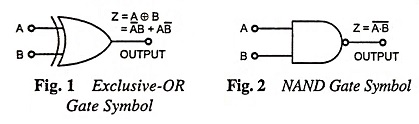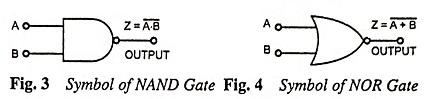## Logic Gates and Boolean Algebra Interview Questions and Answers:

1. What is Boolean algebra?

Ans. Boolean algebra is a mathematical system of logic in which truth functions are expressed as symbols and then these symbols are manipulated to arrive at conclusion.

2. What are the basic logic elements?

Ans. Basic logic elements are NOT gate, AND gate, OR gate and the flip-flop.

3. What is a truth table?

Ans. Truth table is a table that gives outputs for all possible combinations of inputs to a logic circuit.

4. Define positive logic and negative logic.

Ans. If the higher of the two voltages represents a 1 and the lower voltage represents a 0, the logic is called a positive logic. On the other hand, if the lower voltage represents a 1 and the higher voltage a 0, we have a negative logic.

5. What is pulse logic system?

Ans. A logic system in which a bit is recognized by the presence or absence of a pulse is called a pulse or dynamic logic system.

6. What is an inverter?

Ans. An inverter is a logic gate whose output is the inverse or complement of its input.

7. What are the universal logic gates?

Ans. Universal gate is a gate that can perform all the basic logical operations such as NAND and NOR gates.

8. What are basic logic gates and what are universal logic gates?

Ans. Basic logic gates are AND gate, OR gate, and NOT (INVERTER) gate. Universal logic gates are NAND and NOR gates.

9. Draw symbols of EX-OR and NAND gate.

Ans. The symbols of EX-OR and NAND gate are shown below in Fig. 1 and 2 respectively.10. What is the specialty of NAND and NOR gates?

Ans. The specialty of NAND and NOR gates is that they are universal gates and can perform all the basic logical operations.

11. Why NAND-NAND realization is preferred over AND-OR realization?

Ans. NAND-NAND realization needs only one type of gate (NAND), that minimizes IC package counter.

12. Why is a two-input NAND gate called universal gate?

Ans. NAND gate is called universal gate because any digital system can be implemented with the NAND gate. Sequential and combinational circuits can be constructed with these gates because element circuits like flip-flop can be constructed from two NAND gates connected back-to-back. NAND gates are common in hardware because they are easily available in the ICs form. A NAND gate is in fact a NOT-AND gate. It can be obtained by connecting a NOT gate in the output of an AND gate.

13. What is XOR gate ?

Ans. XOR gate is an inequality comparator or detector and produces output only when the inputs are different. It means that XOR gate is a logic gate that produces high output when the two inputs are different (one is high and the other is low) and low output when the two inputs are the same (either low or high).

14. Draw symbols of NAND gate and NOR gate.

Ans. Symbols of NAND gates and NOR gates are given below.15. What is associate law?

Ans. Associate law is a law of addition and multiplication and according to this law grouping of the variables in the ORing or ANDing of several variables is immaterial and the results obtained are the same.

16. Distinguish between sum-of-products and product-of-sums.

Ans. Sum-of-products (SOP) is a logic expression in the form of ANDed terms ORed together where as product-of-sums (POS) is a logic expression in the form of ORed terms ANDed together.

17. What is a minterm?

Ans. A term containing literals corresponding to all the variables in ANDed form is known as a minterm.

18. What are maxterms?

Ans. A term containing literals corresponding to all the variables in ORed form is known as a maxterm.

19. How many output functions are possible when we have n input variables?

Ans. For n binary variables, we can have 2n distinct minterms. Since the function can be either 0 or 1 for each minterm, and since there are 2n minterms, one can calculate the possible functions that can be formed with n variables to be 2n. Thus, for n = 3

Number of minterms = 2n = 23 = 8

and number of possible functions = 2·2n = 2 x 23 = 16.

20. What is the difference between canonical form and standard form?

Ans. In canonical form, a Boolean function is expressed as sum of minterms or product of maxterms and is obtained by reading a function from the truth table. Canonical forms may or may not contain the least numbers of literals because by definition, each maxterm or minterm must contain all variables, complemented or uncomplemented. In standard form, the terms of function may contain one, two or any number of literals. Standard form is expressed as sum of products or product of sums.

21. What is the use of De Morgan’s theorem?

Ans. De Morgan’s theorems are extremely useful in simplification of Boolean expressions in which a sum or product of variables is inverted.

22. What is Karnaugh map?

Ans. Kamaugh map is essentially a geometrical pattern of squares that carry the labels of the logic variables in both normal and complemented forms.

23. What is the use of a Kamaugh map?

Ans. Kamaugh map is used for simplification and manipulation of Boolean expressions.

24. State the limitation of Karnaugh’s map?

Ans. The limitation of Karnaugh’s map is that beyond 6 variables it becomes very cumbersome and so it is generally used up to 6 variables.

Scroll to Top